## 1. Introduction

As an alternative method to welding, riveting and other conventional fasteners, applications of adhesive joints have been increased in structures and industries such as aircraft and automobile . In an assembly of composite structures, usage of mechanical fasteners causes high stress concentration that results in structurally inefficient joints. On the other hand, adhesive joints are capable of redistributing the loads so that stress concentrations can be lowered . Adhesive joints provide good strength–weight and cost-effectiveness ratios. These requirements cannot be met by mechanical joints .

Recently, interest in using artificial neural networks (ANNs) for forecasting has led to huge increase in research areas . Experimental studies are typically time consuming and costly processes and sometimes even complex to perform. To be able to overcome these problems, various modeling techniques are used. ANNs are one of the most popular techniques which get inspired from biological neural networks. The basic units of ANNs are the neurons which are connected to each other with weights. They can be trained to perform a particular function by adjusting the values of these weights . As an effective prediction tool, ANN was frequently used by researchers.

Bardak et al.  used ANN to estimate bonding strength of wood joints pressed under different conditions. Their study showed that model predicted bonding strength with acceptable accuracy. Tiryaki and Hamzaçebi  predicted modulus of rupture (MOR) and modulus of elasticity (MOE) of heat treated woods by using ANN. They compared the actual values of MOR and MOE with the outputs of ANN model and concluded that ANN is successful in estimation of them. Ayatollahi and Akhavan-Safar  proposed a new failure criterion in order to predict the static strength of single lap adhesive joints under tensile loading. Their predictions based on the proposed criterion have good agreements with the experimental data. Tiryaki and Aydın  modeled an ANN structure to estimate compression strength parallel to grain of heat treated woods. With this model, there will be no need to comprehensive experiments. They suggested that, with the usage of ANN, satisfactory results can be obtained which reduces the testing time and cost.

In this study, the failure load in single lap adhesive joints subjected to tensile loading was estimated by using ANN technique. The results showed that ANN model has reliable prediction capability and ANN results are in a very good agreement with the experimental data.

## 2. Materials and method

### 2.1. Experimental detail

The experimental data values in Table 1, width (W) and length of bond area (L) in single lap adhesive joints and failure load under tensile loading are the values used for the training of the ANN. These values were taken from an experimental work of Gültekin et al. . In the experimental study, as adhesive was chosen a two-part epoxy DP460 produced by 3M (St. Paul, MN, USA). AA2024-T3 aluminum alloy was used as adherend. Thickness of adherend and bond is 5 and 0.1 mm, respectively. The geometry of the model is shown in Fig. 1.

Table 1. Average maximum failure loads and displacement values.
Data no W L Failure load Data no W L Failure load
1 25 25 9094 21 25 15 5177
2 20 25 5381 22 20 15 4692
3 15 25 4311 23 15 15 3565
4 10 25 3980 24 10 15 2160
5 5 25 1827 25 5 15 1015
6 25 25 9094 26 15 25 4311
7 25 20 6390 27 15 20 4048
8 25 15 5177 28 15 15 3565
9 25 10 5039 29 15 10 2695
10 25 5 2507 30 15 5 1679
11 25 20 6390 31 25 10 5039
12 20 20 5006 32 20 10 3709
13 15 20 4048 33 15 10 2695
14 10 20 2903 34 10 10 1601
15 5 20 1371 35 5 10 596
16 20 25 5381 36 10 25 3980
17 20 20 5006 37 10 20 2903
18 20 15 4692 38 10 15 2160
19 20 10 3709 39 10 10 1601
20 20 5 2055 40 10 5 1102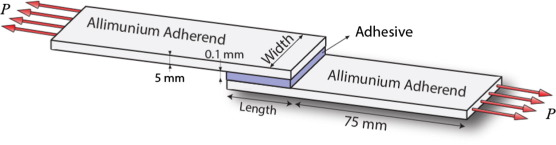Figure 1. Dimensions of a single lap joint.

The experiments of specimen were performed using Shimadzu AG-IS 100 (Shimadzu Corporation, Tokyo, Japan) (100 kN) machine at room temperature and relative humidity 30. During tensile tests, the crosshead speeds were maintained at 1 mm/min .

### 2.2. Artificial neural network

Inspiration of artificial neural network comes from biological neural networks. Basic unit of a biological network is called as neuron and it has four basic components: dendrites, soma, axon, and synapses which receive input signals from the outside and combines them to perform generally nonlinear operation on the result . A typical biological neuron was shown in Fig. 2.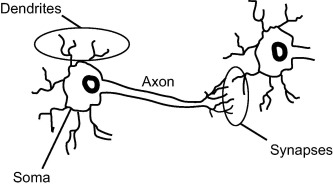Figure 2. A typical biological neuron.

ANN that consists of interconnected processing unit can be considered as highly simplified model of the structure of biological neural network . ANN is very powerful tool for solving complicated engineering problems . Instead of complex rules and mathematical formulations, ANNs are capable of learning key information patterns within a multi-information domain . A simple ANN consists of three layers: input, hidden and output layer. Connection between layers is achieved by weights and they indicate the strength of the connection .

Haykin stated mathematically that, we can describe a neuron k by the following equations :

 $u_{k}=\sum _{j=1}^{m}w_{kj}x_{j}$
(1)
 $y_{k}=\varphi (u_{k}+b_{k})$
(2)

Bias, denoted by bk, has the effect of increasing or lowering the net input of the activation function. x1, x2, … , xm are the inputs; wk1, wk2, … , wkm are the weights of the neuron k; uk   is the linear combiner output due to input signals; ${\textstyle \varphi (.)}$ is the activation function; yk is the output signal of the neuron.

A typical block diagram of model of a neuron is shown in Fig. 3.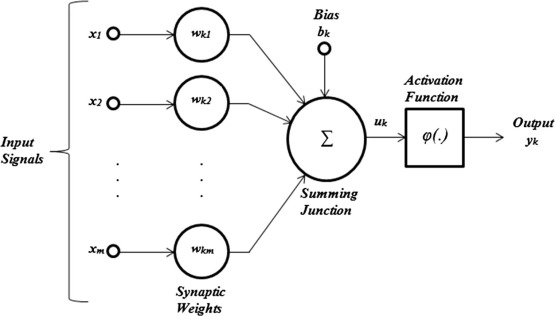Figure 3. Block diagram of model of a neuron .

## 3. Results and discussions

### 3.1. ANN results

In this study, input layer with two neuron, hidden layer with five neurons and output layer with one neuron ANN architecture were used (2-5-1). Data were divided into two parts: training and testing. 88% of data were used to train the network and remaining 12% was used to test the performance of network. Test data were written with bold fonts in Table 1.

Weight and bias values between input and hidden layer was shown in Table 2.

Table 2. Weight and bias values between input and hidden layer for failure load prediction.
i W1i W2i i bi
1 54.98314 −2.41357 1 −41.7572
2 −6.94778 −2.37484 2 14.60742
3 −2.04793 −5.50244 3 3.344352
4 −95.247 46.92676 4 −36.351
5 2.070685 5.488765 5 −3.34514

A three-layer feedforward ANN model was constructed and a backpropagation algorithm was used in order to train network. Levenberg–Marquardt (LM) algorithm was selected as learning algorithm in the present study. Several combinations of transfer functions for hidden and output layer were tried and it was concluded that logistic sigmoid transfer function (logsig) and linear transfer function (purelin) gave best results in the hidden layer and output layer of the network as an activation function, respectively. Since there is no certain rule to decide number of hidden layer neurons, various combinations were tried. After trial and error procedure, best results with minimum errors were obtained with 2-5-1 neuron structure for input, hidden and output layer, respectively.

Since normalizing of the training inputs generally improves the quality of the training , numerical values were normalized by the following formula:

 $x_{i}={\frac {x-x_{min}}{x_{max}-x_{min}}}$
(3)

where ${\textstyle x}$, ${\textstyle x_{i}}$, ${\textstyle x_{min}}$ and ${\textstyle x_{max}}$ are original, normalized, minimum and maximum values, respectively.

Illustration of ANN can be seen in the following Fig. 4.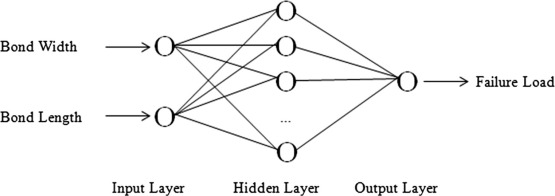Figure 4. Simple model of ANN.

Bond width (W) and bond length (L) were used as input neuron to predict failure load in the network. Prediction equation of failure load was given below:

 ${\mbox{Failure load}}=-0.2202{_{\ast }}F_{1}-152.3947{_{\ast }}F_{2}+$$76.2188{_{\ast }}F_{3}-0.1559{_{\ast }}F_{4}+76.66{_{\ast }}F_{5}+$$76.184$
(4)

Sigmoid function was used to calculate each F values.

 $F_{i}={\frac {1}{1+e^{-E_{i}}}}$
(5)
 $E_{i}=W_{1i}{_{\ast }}W+W_{2i}{_{\ast }}L+b_{1i}$
(6)

Fig. 5 shows results obtained for training (a) and testing (b) period from the ANN model. As seen from the figures, predicted values were compared with experimental values and they are in good agreement and correlation.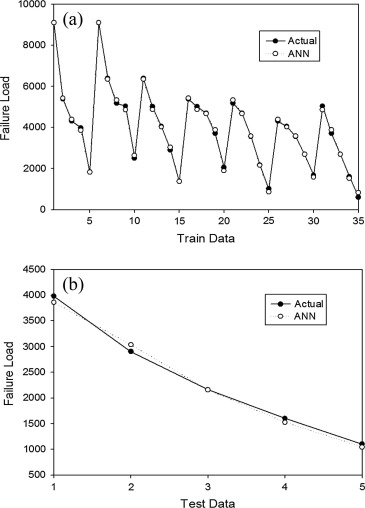Figure 5. (a) Training and (b) testing period of ANN.

### 3.2. Performance of ANN

Various performance parameters can be used to evaluate the convergency of experimental values to predict values. Mean absolute error (MAE), mean absolute percentage error (MAPE), root mean-square error (RMSE) and correlation coefficient (R) were used to study the convergence between the target values and the output values .

In this study, MAPE, Nash–Sutcliffe coefficient of efficiency (NS) and R2 were used as performance parameters. R2 values can clearly be seen from Fig. 6.

 ${\mbox{MAPE}}={\frac {1}{n}}\sum _{i=1}^{n}{\frac {\vert o_{i}-t_{i}\vert }{t_{i}}}\cdot 100$
(7)
 ${\mbox{NS}}=1-{\frac {\sum {\left(t_{i}-o_{i}\right)}^{2}}{\sum {\left(t_{i}-{\overline {t_{i}}}\right)}^{2}}}$
(8)

where ${\textstyle t}$ is target value, ${\textstyle {\overline {t_{i}}}}$ is mean of target, ${\textstyle o}$ is output value and ${\textstyle n}$ is total number of data.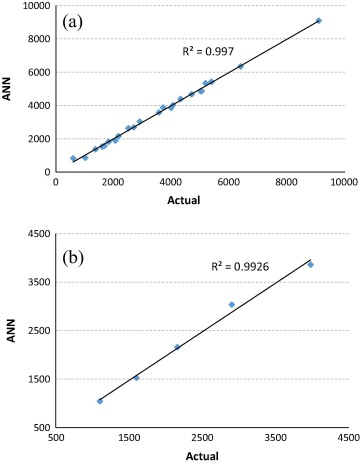Figure 6. Correlation between actual (experimental) and ANN results for (a) training and (b) testing period.

NS can get negative or positive values with a maximum absolute value of 1. A positive value indicates that the predicted values describe the trend of the measured data better than the mean of the actual values, whereas a negative value indicates that the corresponding model output is dissimilar to the behavior of the studied system .

MAPE values (%) and NS values of both training and testing data are 3.523 and 3.524 and 0.997 and 0.992, respectively. As seen from the results that, ANN showed good prediction performance for both training and testing periods.

## 4. Conclusion

In the present study, an ANN model was developed in order to predict failure load of single lap adhesive joints. In training period, LM learning algorithm was used. The best results were obtained in the ANN structure of 2-5-1. In model, R2 values were 0.997 and 0.9926 for training and testing periods, respectively. On the other hand, MAPE (%) values of both training and testing data were 3.523 and 3. 524, respectively. NS values were 0.997 for training period and 0.992 for testing period. Estimated results were within acceptable error limits. All these show that the learning ability of ANN for estimation is very good and using of this method can strongly be suggested to eliminate complicated and time consuming experimental laboratory works.

### Document informationPublished on 12/04/17

Licence: Other

### Document Score0

Views 33
Recommendations 0